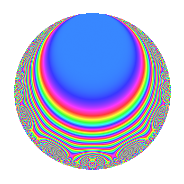# Properties

 Label 402.2.aLevel 402 Weight 2 Character orbit a Rep. character $$\chi_{402}(1,\cdot)$$ Character field $$\Q$$ Dimension 11 Newforms 7 Sturm bound 136 Trace bound 5

# Learn more about

## Defining parameters

 Level: $$N$$ = $$402 = 2 \cdot 3 \cdot 67$$ Weight: $$k$$ = $$2$$ Character orbit: $$[\chi]$$ = 402.a (trivial) Character field: $$\Q$$ Newforms: $$7$$ Sturm bound: $$136$$ Trace bound: $$5$$ Distinguishing $$T_p$$: $$5$$, $$7$$

## Dimensions

The following table gives the dimensions of various subspaces of $$M_{2}(\Gamma_0(402))$$.

Total New Old
Modular forms 72 11 61
Cusp forms 65 11 54
Eisenstein series 7 0 7

The following table gives the dimensions of the cuspidal new subspaces with specified eigenvalues for the Atkin-Lehner operators and the Fricke involution.

$$2$$$$3$$$$67$$FrickeDim.
$$+$$$$+$$$$+$$$$+$$$$1$$
$$+$$$$+$$$$-$$$$-$$$$2$$
$$+$$$$-$$$$+$$$$-$$$$1$$
$$+$$$$-$$$$-$$$$+$$$$1$$
$$-$$$$+$$$$+$$$$-$$$$3$$
$$-$$$$-$$$$-$$$$-$$$$3$$
Plus space$$+$$$$2$$
Minus space$$-$$$$9$$

## Trace form

 $$11q$$ $$\mathstrut +\mathstrut q^{2}$$ $$\mathstrut -\mathstrut q^{3}$$ $$\mathstrut +\mathstrut 11q^{4}$$ $$\mathstrut +\mathstrut 6q^{5}$$ $$\mathstrut +\mathstrut q^{6}$$ $$\mathstrut +\mathstrut 4q^{7}$$ $$\mathstrut +\mathstrut q^{8}$$ $$\mathstrut +\mathstrut 11q^{9}$$ $$\mathstrut +\mathstrut O(q^{10})$$ $$11q$$ $$\mathstrut +\mathstrut q^{2}$$ $$\mathstrut -\mathstrut q^{3}$$ $$\mathstrut +\mathstrut 11q^{4}$$ $$\mathstrut +\mathstrut 6q^{5}$$ $$\mathstrut +\mathstrut q^{6}$$ $$\mathstrut +\mathstrut 4q^{7}$$ $$\mathstrut +\mathstrut q^{8}$$ $$\mathstrut +\mathstrut 11q^{9}$$ $$\mathstrut +\mathstrut 6q^{10}$$ $$\mathstrut +\mathstrut 4q^{11}$$ $$\mathstrut -\mathstrut q^{12}$$ $$\mathstrut -\mathstrut 10q^{13}$$ $$\mathstrut -\mathstrut 2q^{15}$$ $$\mathstrut +\mathstrut 11q^{16}$$ $$\mathstrut -\mathstrut 2q^{17}$$ $$\mathstrut +\mathstrut q^{18}$$ $$\mathstrut -\mathstrut 4q^{19}$$ $$\mathstrut +\mathstrut 6q^{20}$$ $$\mathstrut -\mathstrut 4q^{21}$$ $$\mathstrut +\mathstrut 4q^{22}$$ $$\mathstrut +\mathstrut 12q^{23}$$ $$\mathstrut +\mathstrut q^{24}$$ $$\mathstrut +\mathstrut 25q^{25}$$ $$\mathstrut +\mathstrut 14q^{26}$$ $$\mathstrut -\mathstrut q^{27}$$ $$\mathstrut +\mathstrut 4q^{28}$$ $$\mathstrut +\mathstrut 2q^{29}$$ $$\mathstrut +\mathstrut 2q^{30}$$ $$\mathstrut -\mathstrut 12q^{31}$$ $$\mathstrut +\mathstrut q^{32}$$ $$\mathstrut +\mathstrut 4q^{33}$$ $$\mathstrut +\mathstrut 2q^{34}$$ $$\mathstrut -\mathstrut 8q^{35}$$ $$\mathstrut +\mathstrut 11q^{36}$$ $$\mathstrut -\mathstrut 2q^{37}$$ $$\mathstrut -\mathstrut 4q^{38}$$ $$\mathstrut -\mathstrut 14q^{39}$$ $$\mathstrut +\mathstrut 6q^{40}$$ $$\mathstrut -\mathstrut 6q^{41}$$ $$\mathstrut +\mathstrut 4q^{42}$$ $$\mathstrut -\mathstrut 4q^{43}$$ $$\mathstrut +\mathstrut 4q^{44}$$ $$\mathstrut +\mathstrut 6q^{45}$$ $$\mathstrut -\mathstrut 20q^{47}$$ $$\mathstrut -\mathstrut q^{48}$$ $$\mathstrut -\mathstrut 9q^{49}$$ $$\mathstrut -\mathstrut q^{50}$$ $$\mathstrut -\mathstrut 10q^{51}$$ $$\mathstrut -\mathstrut 10q^{52}$$ $$\mathstrut +\mathstrut 22q^{53}$$ $$\mathstrut +\mathstrut q^{54}$$ $$\mathstrut -\mathstrut 24q^{55}$$ $$\mathstrut -\mathstrut 12q^{57}$$ $$\mathstrut +\mathstrut 10q^{58}$$ $$\mathstrut -\mathstrut 24q^{59}$$ $$\mathstrut -\mathstrut 2q^{60}$$ $$\mathstrut -\mathstrut 26q^{61}$$ $$\mathstrut -\mathstrut 24q^{62}$$ $$\mathstrut +\mathstrut 4q^{63}$$ $$\mathstrut +\mathstrut 11q^{64}$$ $$\mathstrut -\mathstrut 12q^{65}$$ $$\mathstrut -\mathstrut 12q^{66}$$ $$\mathstrut +\mathstrut q^{67}$$ $$\mathstrut -\mathstrut 2q^{68}$$ $$\mathstrut -\mathstrut 16q^{69}$$ $$\mathstrut -\mathstrut 32q^{70}$$ $$\mathstrut -\mathstrut 28q^{71}$$ $$\mathstrut +\mathstrut q^{72}$$ $$\mathstrut +\mathstrut 34q^{73}$$ $$\mathstrut +\mathstrut 14q^{74}$$ $$\mathstrut -\mathstrut 15q^{75}$$ $$\mathstrut -\mathstrut 4q^{76}$$ $$\mathstrut -\mathstrut 16q^{77}$$ $$\mathstrut -\mathstrut 14q^{78}$$ $$\mathstrut -\mathstrut 20q^{79}$$ $$\mathstrut +\mathstrut 6q^{80}$$ $$\mathstrut +\mathstrut 11q^{81}$$ $$\mathstrut -\mathstrut 6q^{82}$$ $$\mathstrut +\mathstrut 24q^{83}$$ $$\mathstrut -\mathstrut 4q^{84}$$ $$\mathstrut -\mathstrut 12q^{85}$$ $$\mathstrut -\mathstrut 12q^{86}$$ $$\mathstrut +\mathstrut 6q^{87}$$ $$\mathstrut +\mathstrut 4q^{88}$$ $$\mathstrut -\mathstrut 18q^{89}$$ $$\mathstrut +\mathstrut 6q^{90}$$ $$\mathstrut -\mathstrut 24q^{91}$$ $$\mathstrut +\mathstrut 12q^{92}$$ $$\mathstrut -\mathstrut 4q^{93}$$ $$\mathstrut -\mathstrut 32q^{94}$$ $$\mathstrut -\mathstrut 56q^{95}$$ $$\mathstrut +\mathstrut q^{96}$$ $$\mathstrut -\mathstrut 10q^{97}$$ $$\mathstrut -\mathstrut 7q^{98}$$ $$\mathstrut +\mathstrut 4q^{99}$$ $$\mathstrut +\mathstrut O(q^{100})$$

## Decomposition of $$S_{2}^{\mathrm{new}}(\Gamma_0(402))$$ into irreducible Hecke orbits

Label Dim. $$A$$ Field CM Traces A-L signs $q$-expansion
$$a_2$$ $$a_3$$ $$a_5$$ $$a_7$$ 2 3 67
402.2.a.a $$1$$ $$3.210$$ $$\Q$$ None $$-1$$ $$-1$$ $$1$$ $$-3$$ $$+$$ $$+$$ $$+$$ $$q-q^{2}-q^{3}+q^{4}+q^{5}+q^{6}-3q^{7}+\cdots$$
402.2.a.b $$1$$ $$3.210$$ $$\Q$$ None $$-1$$ $$1$$ $$-3$$ $$-1$$ $$+$$ $$-$$ $$-$$ $$q-q^{2}+q^{3}+q^{4}-3q^{5}-q^{6}-q^{7}+\cdots$$
402.2.a.c $$1$$ $$3.210$$ $$\Q$$ None $$-1$$ $$1$$ $$2$$ $$0$$ $$+$$ $$-$$ $$+$$ $$q-q^{2}+q^{3}+q^{4}+2q^{5}-q^{6}-q^{8}+\cdots$$
402.2.a.d $$1$$ $$3.210$$ $$\Q$$ None $$1$$ $$-1$$ $$2$$ $$2$$ $$-$$ $$+$$ $$+$$ $$q+q^{2}-q^{3}+q^{4}+2q^{5}-q^{6}+2q^{7}+\cdots$$
402.2.a.e $$2$$ $$3.210$$ $$\Q(\sqrt{3})$$ None $$-2$$ $$-2$$ $$0$$ $$6$$ $$+$$ $$+$$ $$-$$ $$q-q^{2}-q^{3}+q^{4}+2\beta q^{5}+q^{6}+(3+\cdots)q^{7}+\cdots$$
402.2.a.f $$2$$ $$3.210$$ $$\Q(\sqrt{41})$$ None $$2$$ $$-2$$ $$1$$ $$-1$$ $$-$$ $$+$$ $$+$$ $$q+q^{2}-q^{3}+q^{4}+\beta q^{5}-q^{6}-\beta q^{7}+\cdots$$
402.2.a.g $$3$$ $$3.210$$ 3.3.316.1 None $$3$$ $$3$$ $$3$$ $$1$$ $$-$$ $$-$$ $$-$$ $$q+q^{2}+q^{3}+q^{4}+(1-\beta _{2})q^{5}+q^{6}+\cdots$$

## Decomposition of $$S_{2}^{\mathrm{old}}(\Gamma_0(402))$$ into lower level spaces

$$S_{2}^{\mathrm{old}}(\Gamma_0(402)) \cong$$ $$S_{2}^{\mathrm{new}}(\Gamma_0(67))$$$$^{\oplus 4}$$$$\oplus$$$$S_{2}^{\mathrm{new}}(\Gamma_0(134))$$$$^{\oplus 2}$$$$\oplus$$$$S_{2}^{\mathrm{new}}(\Gamma_0(201))$$$$^{\oplus 2}$$